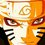# Olympiad Proof Problem - Day 4

Find all primes such that $p^3 - q^7 = p-q$.

Try more proof problems at Olympiad Proof Problems.Note by Surya Prakash
5 years, 7 months ago

This discussion board is a place to discuss our Daily Challenges and the math and science related to those challenges. Explanations are more than just a solution — they should explain the steps and thinking strategies that you used to obtain the solution. Comments should further the discussion of math and science.

When posting on Brilliant:

• Use the emojis to react to an explanation, whether you're congratulating a job well done , or just really confused .
• Ask specific questions about the challenge or the steps in somebody's explanation. Well-posed questions can add a lot to the discussion, but posting "I don't understand!" doesn't help anyone.
• Try to contribute something new to the discussion, whether it is an extension, generalization or other idea related to the challenge.
• Stay on topic — we're all here to learn more about math and science, not to hear about your favorite get-rich-quick scheme or current world events.

MarkdownAppears as
*italics* or _italics_ italics
**bold** or __bold__ bold
- bulleted- list
• bulleted
• list
1. numbered2. list
1. numbered
2. list
Note: you must add a full line of space before and after lists for them to show up correctly
paragraph 1paragraph 2

paragraph 1

paragraph 2

[example link](https://brilliant.org)example link
> This is a quote
This is a quote
    # I indented these lines
# 4 spaces, and now they show
# up as a code block.

print "hello world"
# I indented these lines
# 4 spaces, and now they show
# up as a code block.

print "hello world"
MathAppears as
Remember to wrap math in $$ ... $$ or $ ... $ to ensure proper formatting.
2 \times 3 $2 \times 3$
2^{34} $2^{34}$
a_{i-1} $a_{i-1}$
\frac{2}{3} $\frac{2}{3}$
\sqrt{2} $\sqrt{2}$
\sum_{i=1}^3 $\sum_{i=1}^3$
\sin \theta $\sin \theta$
\boxed{123} $\boxed{123}$

## Comments

Sort by:

Top Newest

Only one pair of primes exist. I used casework to solve this.

We have

$p^3-q^7=p-q$

$p^3-p=q^7-q$

$p(p-1)(p+1)=q(q^3-1)(q^3+1)$

$p(p-1)(p+1)=q(q-1)(q+1)(q^2+q+1)(q^2-q+1) \quad \ldots (1)$

I will first check all cases when $p^3 < q^7$, that is, when $p^3-q^7$ is negative. Note that since $p$ and $q$ are primes, one of $q$, $q-1$, $q+1$, $q^2+q+1$ or $q^2-q+1$ is equal to $p$ assuming $q \neq 2$ (This issue will be addressed).

Since the difference is negative, we must have $p < q$. Combining this with what we know, we have $p=q-1$. (Note: $p \neq q^2 - q + 1$ since the quadratic is greater than $q$ for all positive $q$.) Since $p$ and $q$ are primes, we have $p=2$, $q=3$. Checking, we find that this is incorrect so there are no solutions in this case.

Now, we check when $p = q$. We get that $p-1 = q-1$ and $p+1 = q+1$, rendering $q^2+q+1=1$ and $q^2-q+1=1$, which gives $p=q=0$ which are not primes. There are no solutions in this case.

Finally, we check when $p > q$. We have the following values for $p$: $p=q+1$, $p=q^2-q+1$, $p=q^2+q+1$. We will now look at each expresion for $p$.

Case 1: $p=q+1$

We get that $p=3$, $q=2$. Checking, we find that this is incorrect. Therefore, there are no solutions in this case.

Case 2: $p=q^2-q+1$

We have $p=q^2-q+1$ so $p-1=q^2-q=q(q-1)$ which leaves $p+1=(q+1)(q^2+q+1)$ from $(1)$. We now have

$q^2-q+1+1=(q+1)(q^2+q+1)$

$q^2-q+2=q^3+2q^2+2q+1$

$q^3+q^2+3q-1=0$

Playing with this cubic, we find that it has no integral solutions for $q$ (There are many different ways to check). Hence, there are no solutions in this case.

Case 3: $p=q^2+q+1$

In this case, we have $p=q^2+q+1$, so $p-1=q^2+q=q(q+1)$, which leaves $p+1=(q-1)(q^2-q+1)$ from $(1)$. We now have the following:

$q^2+q+1+1=(q-1)(q^2-q+1)$

$q^2+q+2=q^3-2q^2+2q-1$

$q^3-3q^2+q-3=0$

$(q^2+3)(q-3)=0$

Clearly, only $q=3$ is the integral solution in this case, which implies $p=13$. Checking, we find that this is true. Therefore, only one solution exists in this case: $p=13$, $q=3$.

Thus, the only solution which satisfies is $p=13$, $q=3$.

Great problem, Surya.

- 5 years, 7 months ago

Log in to reply

This is a great problem and solution!

- 5 years, 6 months ago

Log in to reply

Same solution.

- 4 years, 5 months ago

Log in to reply

@Surya Prakash , is this a satisfactory solution?

- 5 years, 7 months ago

Log in to reply

Respected Sharky, I did not understand the second sentence below equation 1 ( Note that since p & q are primes, ...) I request you to explain.

- 5 years, 6 months ago

Log in to reply

Since $p$ and $q$ are primes, they both have 2 factors each: $1$ and the number itself. This implies that $p$ is equivalent to one of the expressions in the brackets.

- 5 years, 6 months ago

Log in to reply

No such pair of primes exist.

- 5 years, 7 months ago

Log in to reply

No. There exists.

- 5 years, 7 months ago

Log in to reply

Check out p=13 and q=3.

- 5 years, 7 months ago

Log in to reply

×

Problem Loading...

Note Loading...

Set Loading...